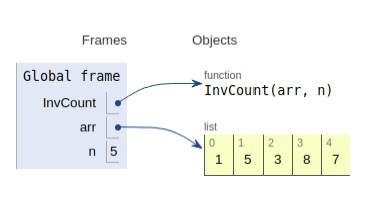# Python Program to Count Inversions in an array

Problem statement − We are given a list, we need to count the inversion required and display it.

Inversion count is obtained by counting how many steps are needed for the array to be sorted.

Now let’s observe the solution in the implementation below −

## Example

Live Demo

# count
def InvCount(arr, n):
inv_count = 0
for i in range(n):
for j in range(i + 1, n):
if (arr[i] > arr[j]):
inv_count += 1
return inv_count
# Driver Code
arr = [1,5,3,8,7]
n = len(arr)
print("Total number of inversions are:",InvCount(arr, n))

## Output

Total number of inversions are: 2All the variables are declared in the local scope and their references are seen in the figure above.

## Conclusion

In this article, we have learned about how we can make a Python Program to Count Inversions in an array

Updated on: 20-Dec-2019

163 Views